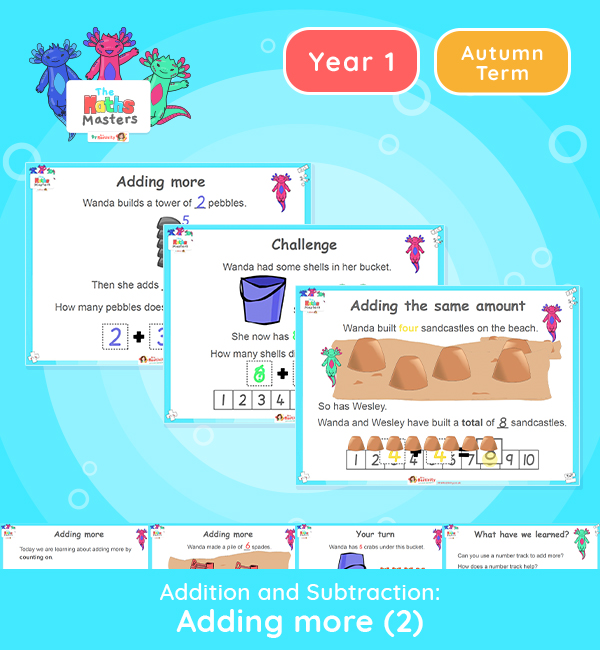# Year 1 | Adding More Part 2 Lesson Presentation## Year 1 addition and subtraction resources

Aligned with the maths mastery approach, this Year 1 | Adding More Part 2 Lesson Presentation is fully editable and designed for the Year 1 maths curriculum to cover the following curriculum objectives:

This lesson is the second of two lessons on adding more using the method of counting on, including use of a number track.

NC Links: Read, write and interpret mathematical statements involving addition (+), and equals (=) signs. • Add one digit numbers to 10, including zero. • Solve one step problems that involve addition using concrete objects and pictorial representations and missing number problems.

Previous Experience: Devise and record number stories, using pictures, numbers and symbols.

TAF Statements: Working Towards Add and subtract (one digit numbers) explaining their method verbally in pictures or using apparatus.

Aligned with the order of teaching of the maths mastery approach, use this to help your pupils get to grips with each mathematical concept. This lesson presentation also includes varied fluency activities, problem solving, and mathematical discussion questions too.

Explore our other year 1 addition and subtraction resources.

## Recently Viewed